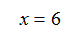Method 2

Another type of log equation is one where there is a log on both sides of the equal sign. To solve this type of equation, use the inverse relationship between logs and exponents.

Example 1 Solve the equation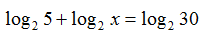Step 1. Use Product of a Power Property to convert to a single log.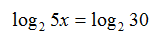Step 2. Use the inverse operation of exponents on both sides of the equals sign. Since the log has a base of 2, use an exponential base of 2.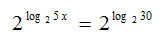Step 3. Since logs and exponents are inverses, the exponent base and the log base cancel.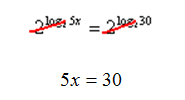Step 4 . Solve for x.